# Selina Solutions Concise Mathematics Class 6 Chapter 8: H.C.F and L.C.M Exercise 8(B)

Selina Solutions Concise Mathematics Class 6 Chapter 8 H.C.F and L.C.M Exercise 8(B) covers questions based on finding H.C.F using common factor, prime factor and division method. The solutions under this exercise, provide an understandable way of solving complex questions present in textbooks. Students who aim to perform very well in the annual exams, are suggested to practice Selina Solutions repeatedly. These Solutions prepared by an expert team, are the best study tool during exam preparation. Students can access the PDF of Selina Solutions Concise Mathematics Class 6 Chapter 8 H.C.F and L.C.M Exercise 8(B), from the links given here

## Selina Solutions Concise Mathematics Class 6 Chapter 8: H.C.F and L.C.M Exercise 8(B) Download PDF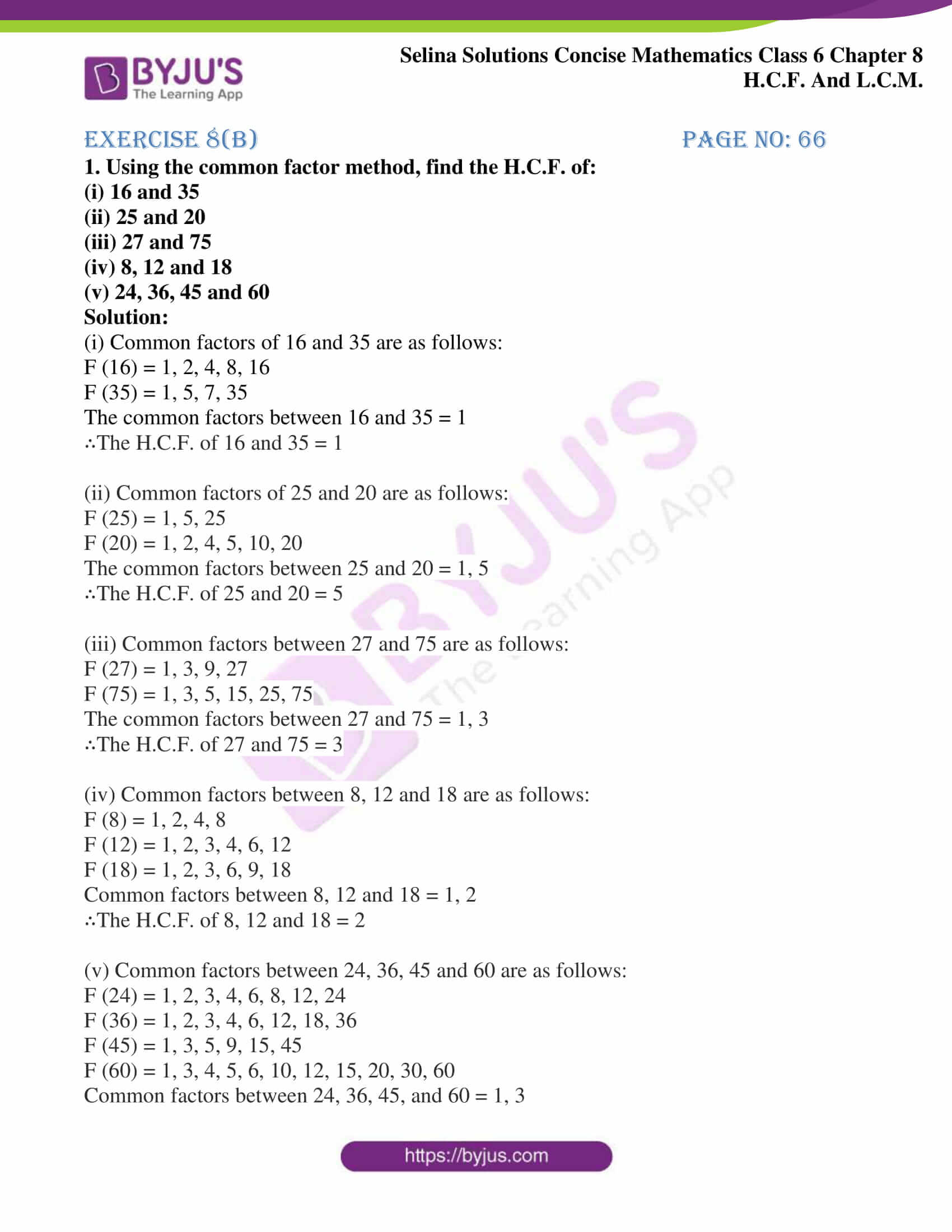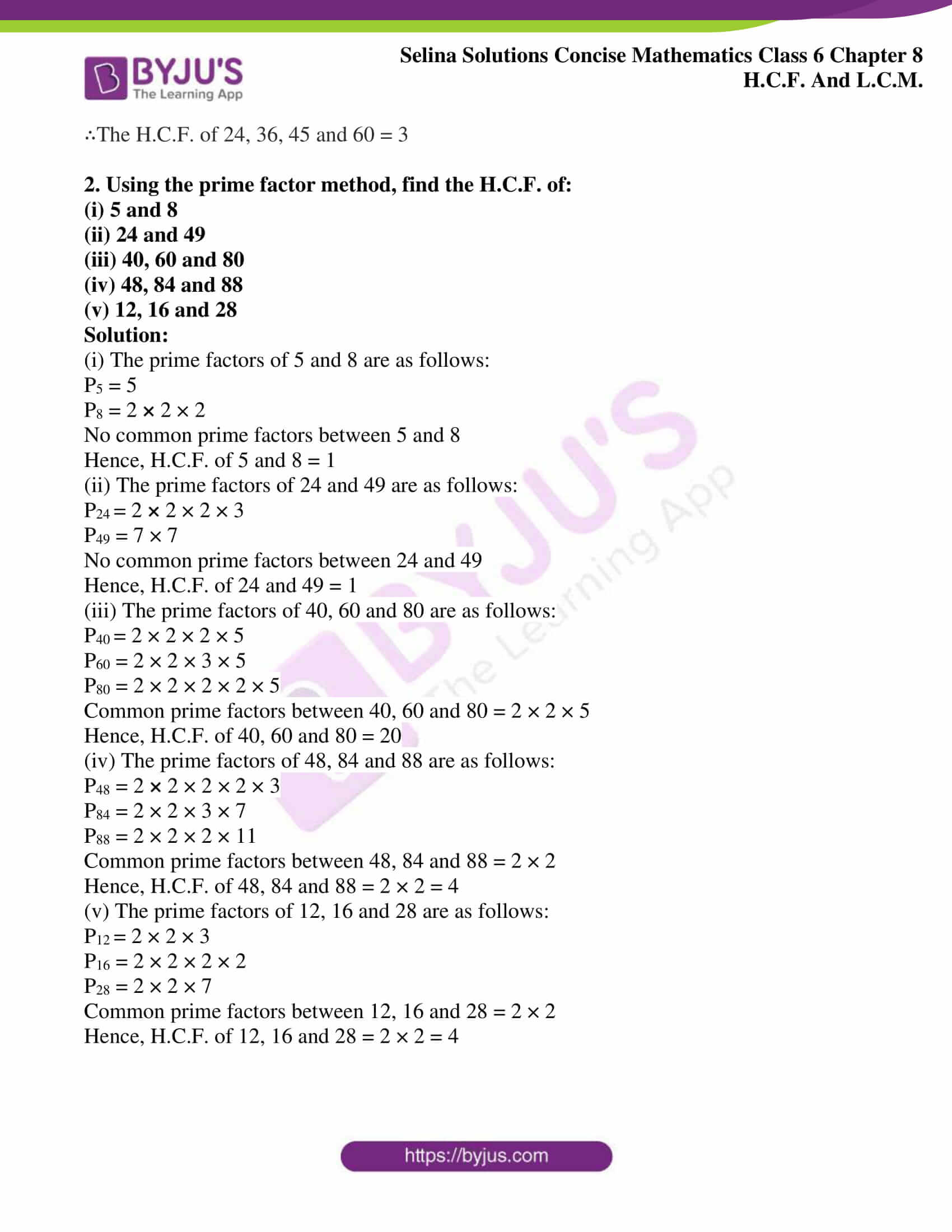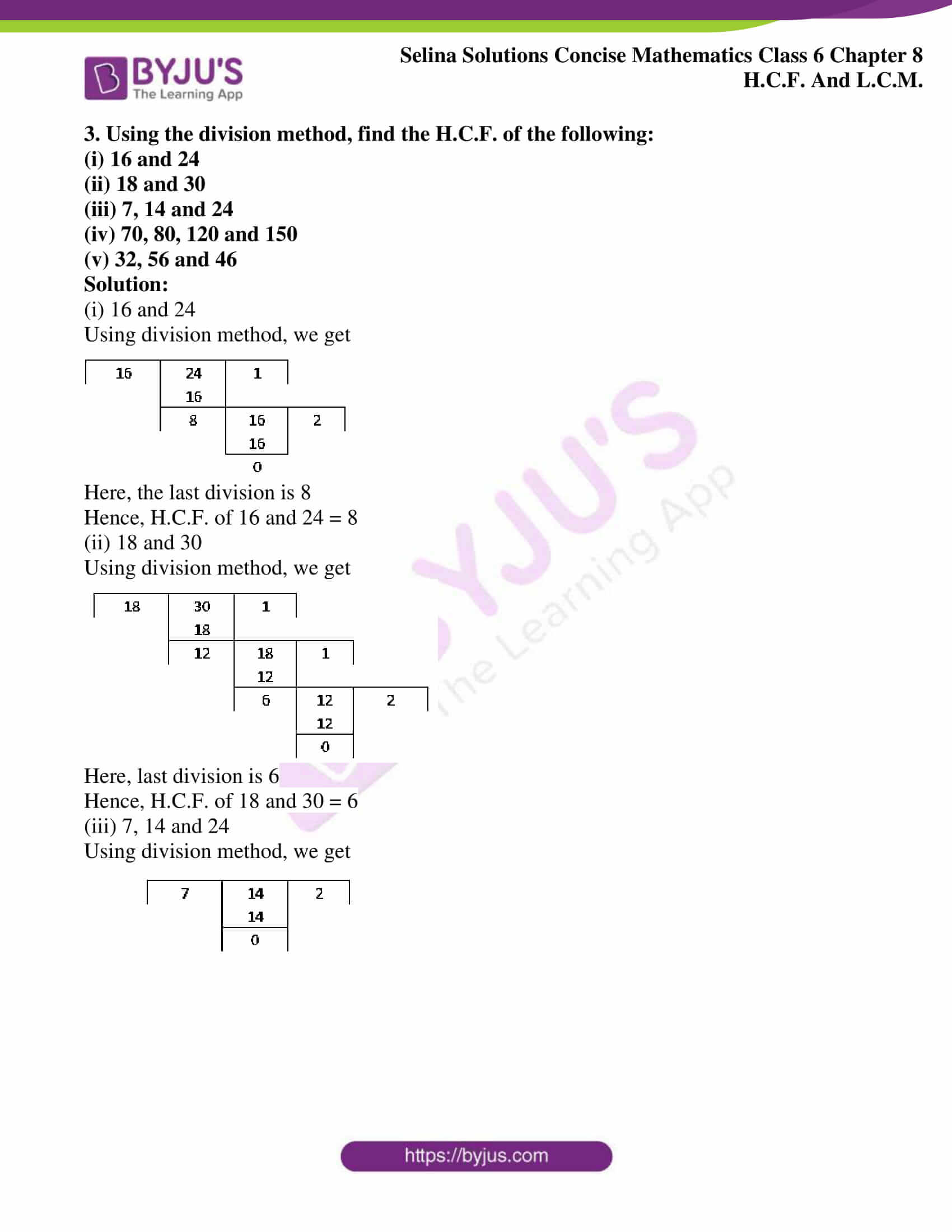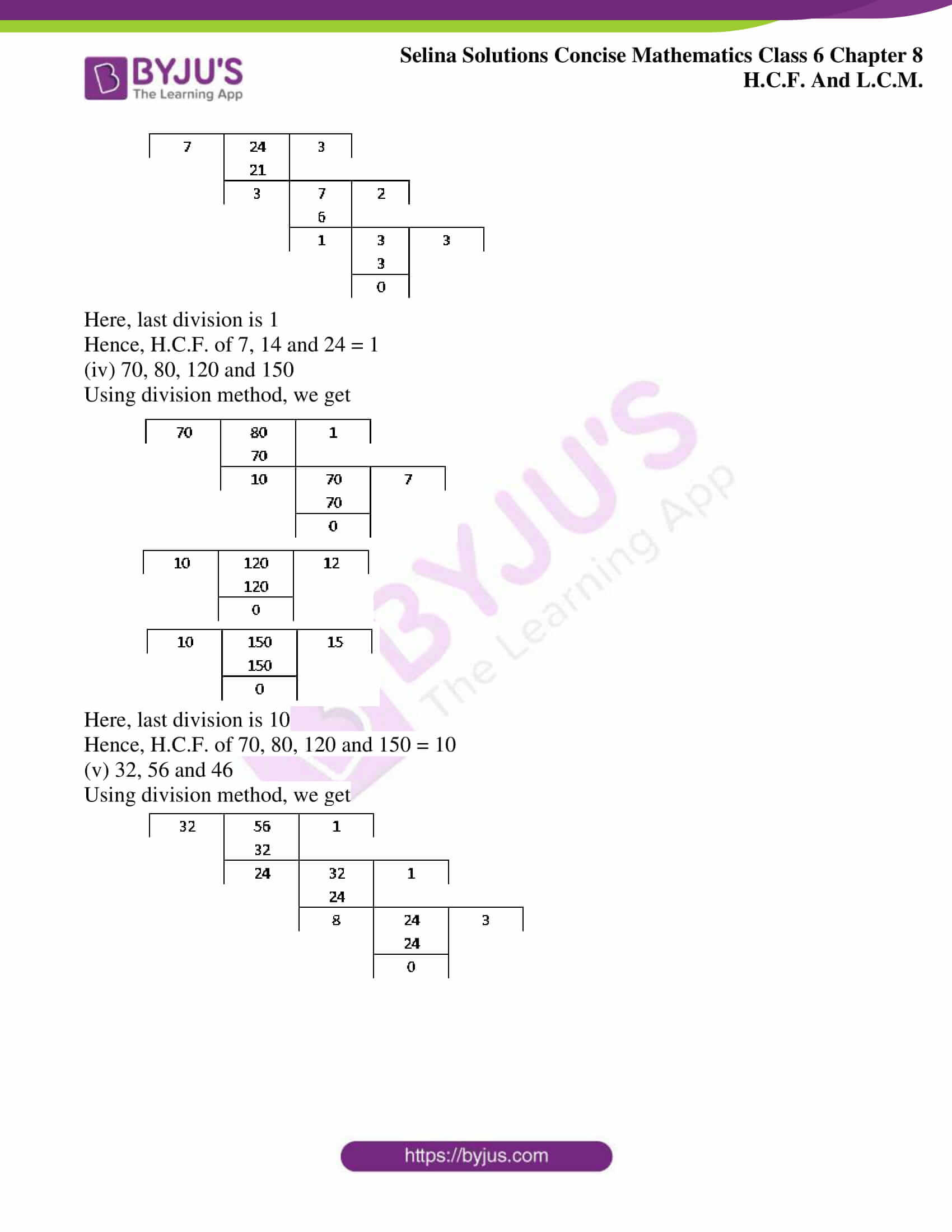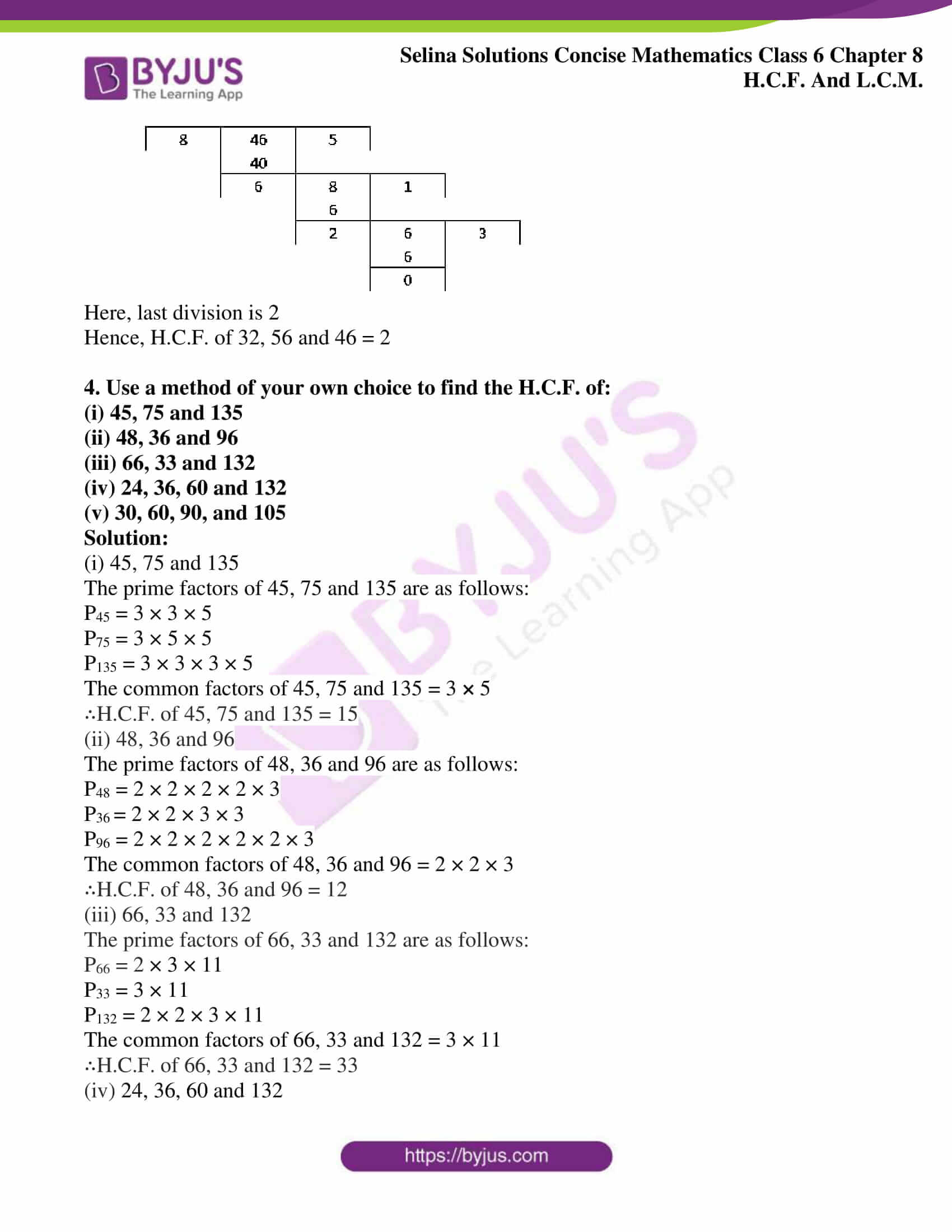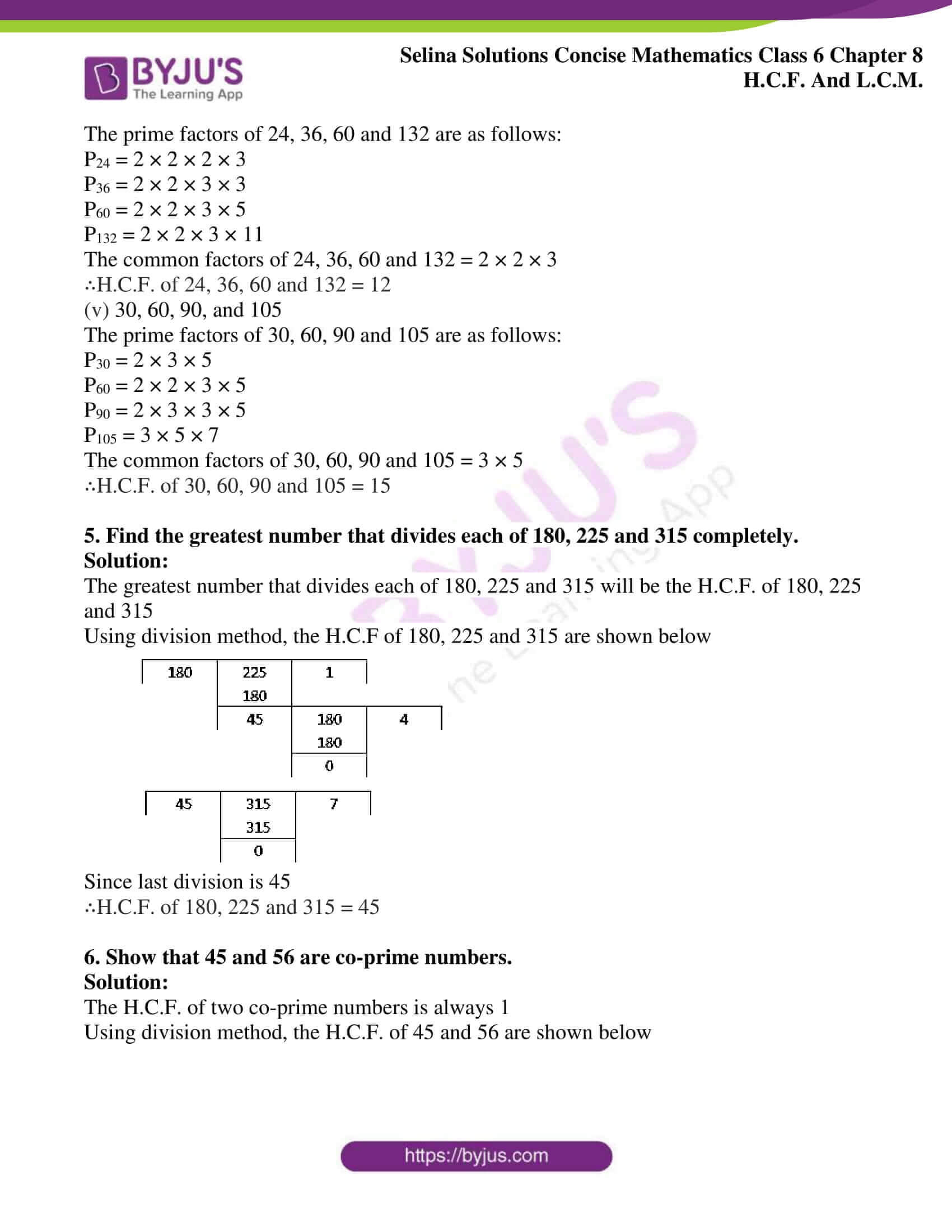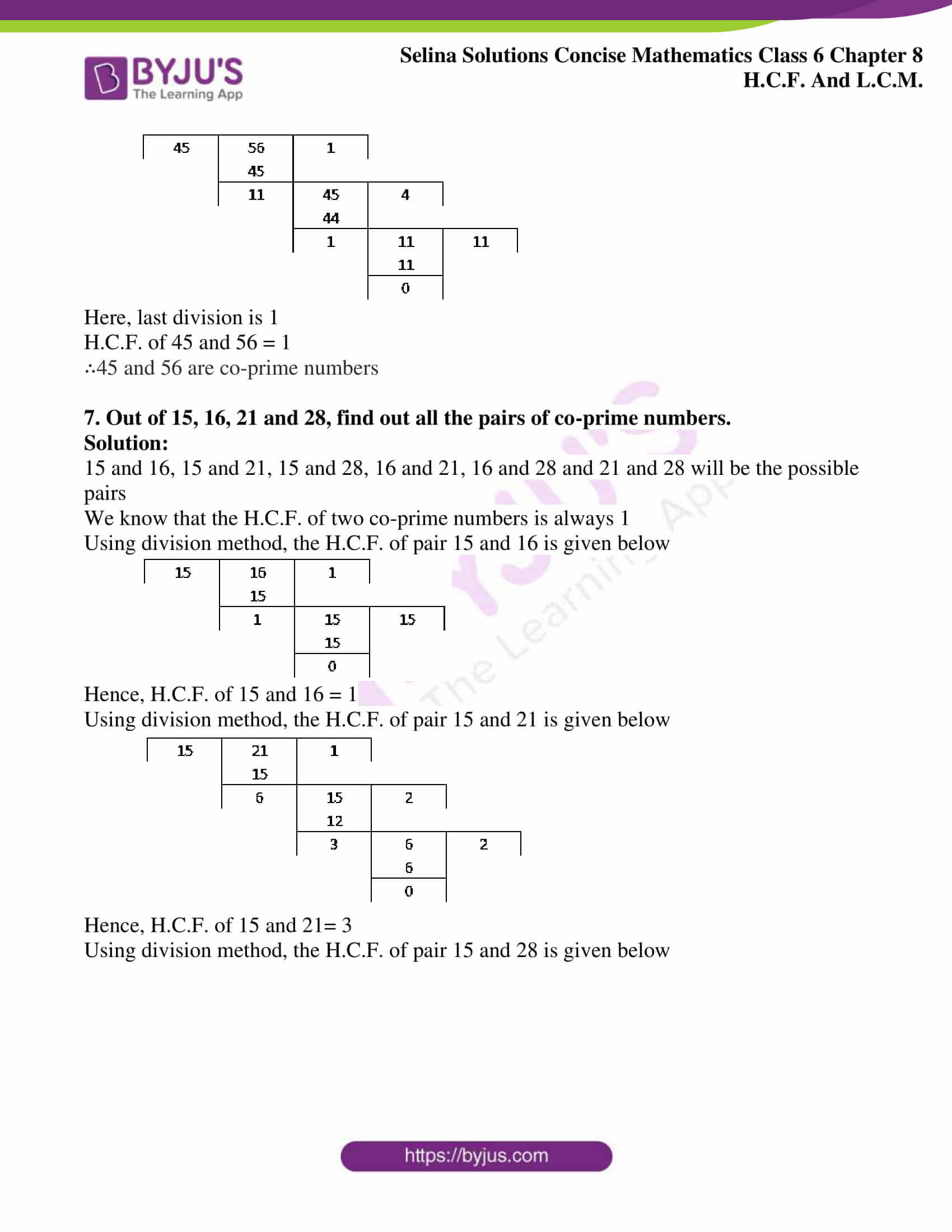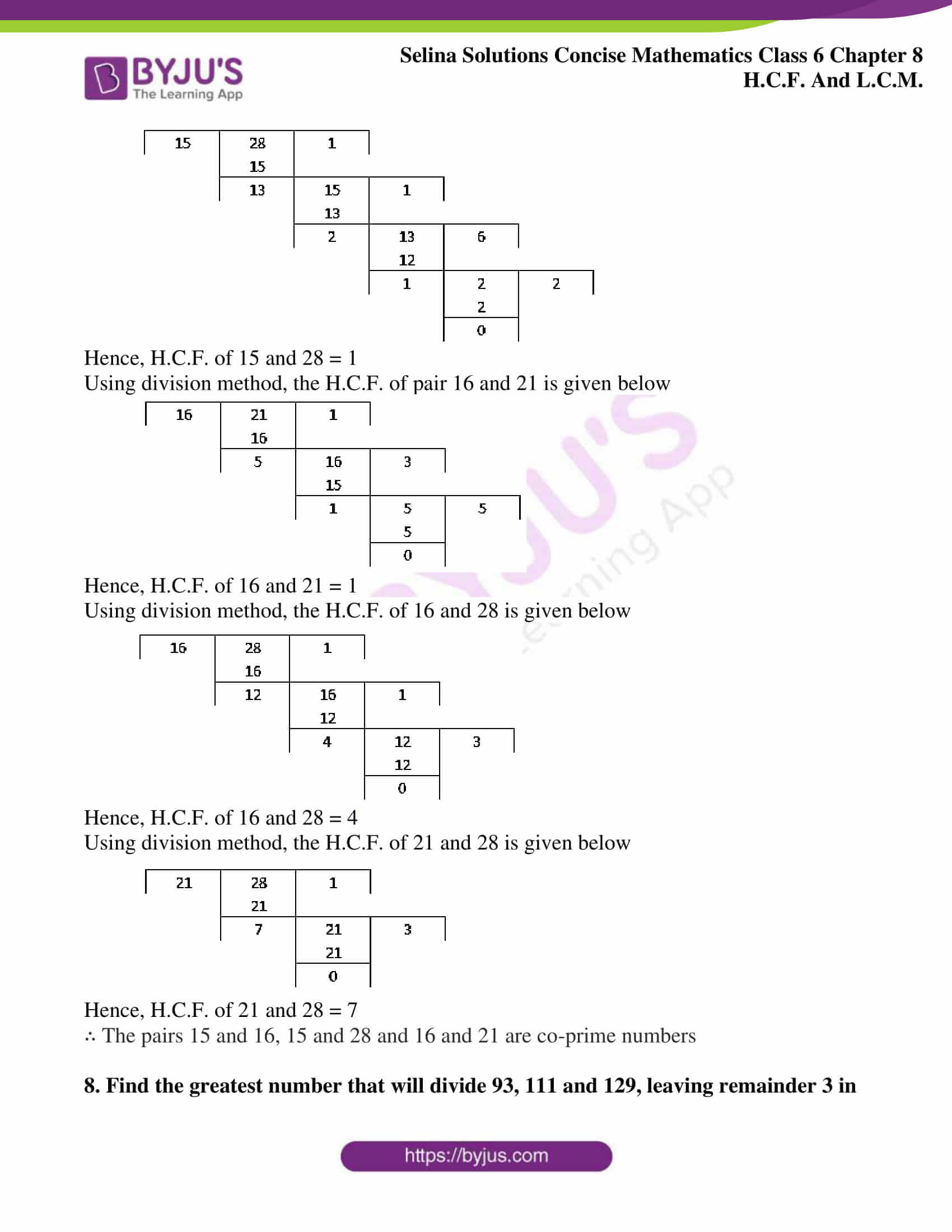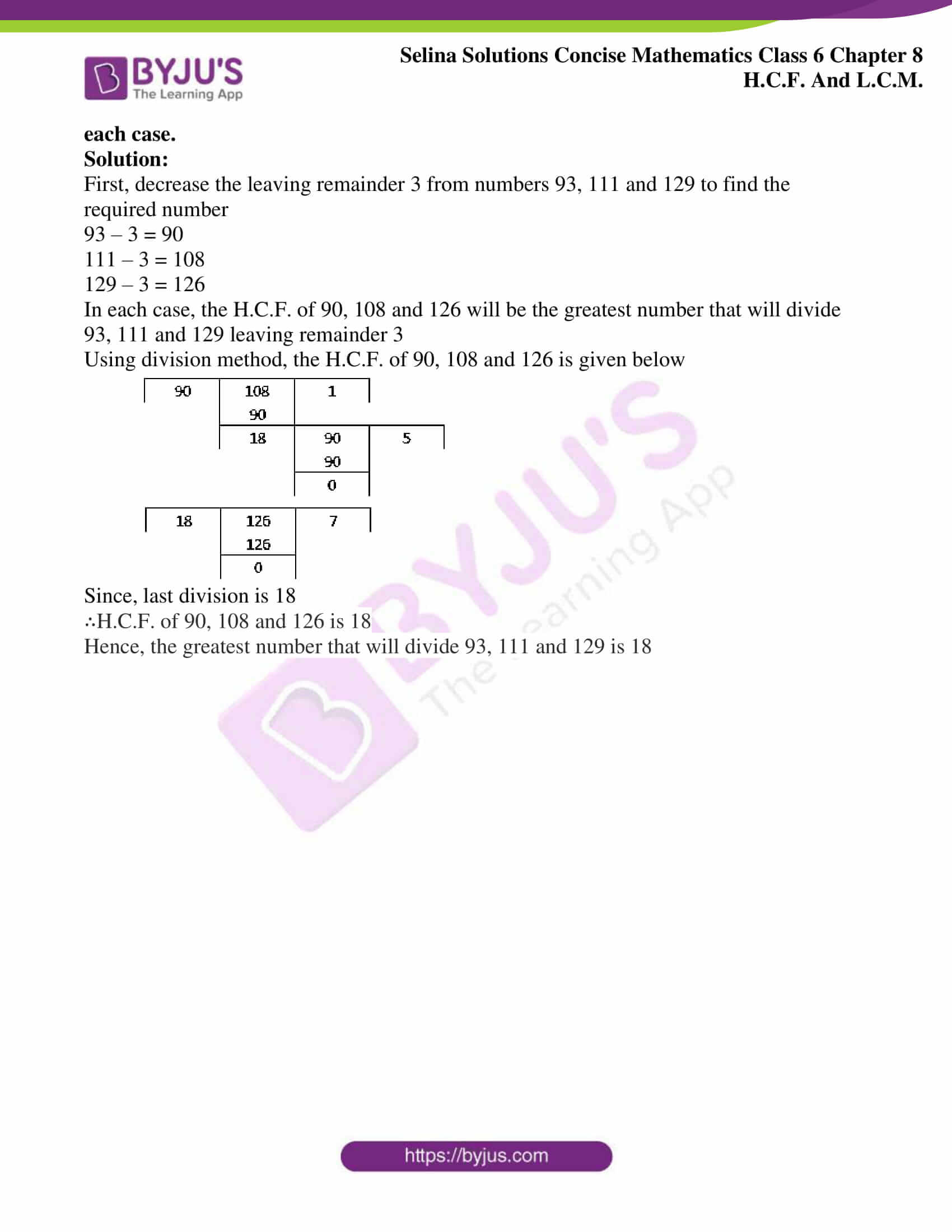### Access other exercises of Selina Solutions Concise Mathematics Class 6 Chapter 8: H.C.F and L.C.M

Exercise 8(A) Solutions

Exercise 8(C) Solutions

### Access Selina Solutions Concise Mathematics Class 6 Chapter 8: H.C.F and L.C.M Exercise 8(B)

#### Exercise 8(B) Page no: 66

1. Using the common factor method, find the H.C.F. of:

(i) 16 and 35

(ii) 25 and 20

(iii) 27 and 75

(iv) 8, 12 and 18

(v) 24, 36, 45 and 60

Solution:

(i) Common factors of 16 and 35 are as follows:

F (16) = 1, 2, 4, 8, 16

F (35) = 1, 5, 7, 35

The common factors between 16 and 35 = 1

∴The H.C.F. of 16 and 35 = 1

(ii) Common factors of 25 and 20 are as follows:

F (25) = 1, 5, 25

F (20) = 1, 2, 4, 5, 10, 20

The common factors between 25 and 20 = 1, 5

∴The H.C.F. of 25 and 20 = 5

(iii) Common factors between 27 and 75 are as follows:

F (27) = 1, 3, 9, 27

F (75) = 1, 3, 5, 15, 25, 75

The common factors between 27 and 75 = 1, 3

∴The H.C.F. of 27 and 75 = 3

(iv) Common factors between 8, 12 and 18 are as follows:

F (8) = 1, 2, 4, 8

F (12) = 1, 2, 3, 4, 6, 12

F (18) = 1, 2, 3, 6, 9, 18

Common factors between 8, 12 and 18 = 1, 2

∴The H.C.F. of 8, 12 and 18 = 2

(v) Common factors between 24, 36, 45 and 60 are as follows:

F (24) = 1, 2, 3, 4, 6, 8, 12, 24

F (36) = 1, 2, 3, 4, 6, 12, 18, 36

F (45) = 1, 3, 5, 9, 15, 45

F (60) = 1, 3, 4, 5, 6, 10, 12, 15, 20, 30, 60

Common factors between 24, 36, 45, and 60 = 1, 3

∴The H.C.F. of 24, 36, 45 and 60 = 3

2. Using the prime factor method, find the H.C.F. of:

(i) 5 and 8

(ii) 24 and 49

(iii) 40, 60 and 80

(iv) 48, 84 and 88

(v) 12, 16 and 28

Solution:

(i) The prime factors of 5 and 8 are as follows:

P5 = 5

P8 = 2 × 2 × 2

No common prime factors between 5 and 8

Hence, H.C.F. of 5 and 8 = 1

(ii) The prime factors of 24 and 49 are as follows:

P24 = 2 × 2 × 2 × 3

P49 = 7 × 7

No common prime factors between 24 and 49

Hence, H.C.F. of 24 and 49 = 1

(iii) The prime factors of 40, 60 and 80 are as follows:

P40 = 2 × 2 × 2 × 5

P60 = 2 × 2 × 3 × 5

P80 = 2 × 2 × 2 × 2 × 5

Common prime factors between 40, 60 and 80 = 2 × 2 × 5

Hence, H.C.F. of 40, 60 and 80 = 20

(iv) The prime factors of 48, 84 and 88 are as follows:

P48 = 2 × 2 × 2 × 2 × 3

P84 = 2 × 2 × 3 × 7

P88 = 2 × 2 × 2 × 11

Common prime factors between 48, 84 and 88 = 2 × 2

Hence, H.C.F. of 48, 84 and 88 = 2 × 2 = 4

(v) The prime factors of 12, 16 and 28 are as follows:

P12 = 2 × 2 × 3

P16 = 2 × 2 × 2 × 2

P28 = 2 × 2 × 7

Common prime factors between 12, 16 and 28 = 2 × 2

Hence, H.C.F. of 12, 16 and 28 = 2 × 2 = 4

3. Using the division method, find the H.C.F. of the following:

(i) 16 and 24

(ii) 18 and 30

(iii) 7, 14 and 24

(iv) 70, 80, 120 and 150

(v) 32, 56 and 46

Solution:

(i) 16 and 24

Using division method, we get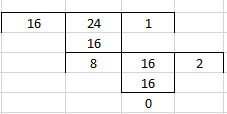Here, the last division is 8

Hence, H.C.F. of 16 and 24 = 8

(ii) 18 and 30

Using division method, we get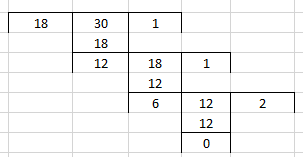Here, last division is 6

Hence, H.C.F. of 18 and 30 = 6

(iii) 7, 14 and 24

Using division method, we get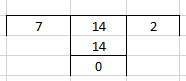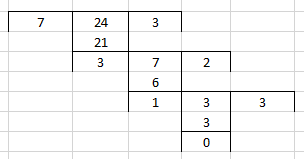Here, last division is 1

Hence, H.C.F. of 7, 14 and 24 = 1

(iv) 70, 80, 120 and 150

Using division method, we get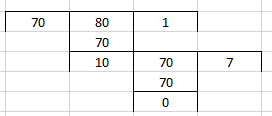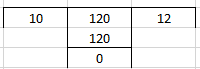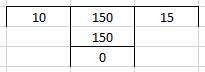Here, last division is 10

Hence, H.C.F. of 70, 80, 120 and 150 = 10

(v) 32, 56 and 46

Using division method, we get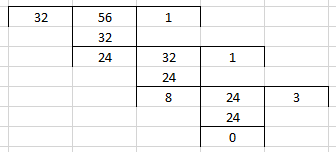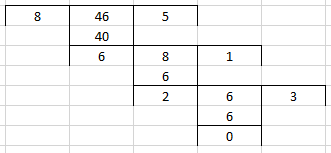Here, last division is 2

Hence, H.C.F. of 32, 56 and 46 = 2

4. Use a method of your own choice to find the H.C.F. of:

(i) 45, 75 and 135

(ii) 48, 36 and 96

(iii) 66, 33 and 132

(iv) 24, 36, 60 and 132

(v) 30, 60, 90, and 105

Solution:

(i) 45, 75 and 135

The prime factors of 45, 75 and 135 are as follows:

P45 = 3 × 3 × 5

P75 = 3 × 5 × 5

P135 = 3 × 3 × 3 × 5

The common factors of 45, 75 and 135 = 3 × 5

∴H.C.F. of 45, 75 and 135 = 15

(ii) 48, 36 and 96

The prime factors of 48, 36 and 96 are as follows:

P48 = 2 × 2 × 2 × 2 × 3

P36 = 2 × 2 × 3 × 3

P96 = 2 × 2 × 2 × 2 × 2 × 3

The common factors of 48, 36 and 96 = 2 × 2 × 3

∴H.C.F. of 48, 36 and 96 = 12

(iii) 66, 33 and 132

The prime factors of 66, 33 and 132 are as follows:

P66 = 2 × 3 × 11

P33 = 3 × 11

P132 = 2 × 2 × 3 × 11

The common factors of 66, 33 and 132 = 3 × 11

∴H.C.F. of 66, 33 and 132 = 33

(iv) 24, 36, 60 and 132

The prime factors of 24, 36, 60 and 132 are as follows:

P24 = 2 × 2 × 2 × 3

P36 = 2 × 2 × 3 × 3

P60 = 2 × 2 × 3 × 5

P132 = 2 × 2 × 3 × 11

The common factors of 24, 36, 60 and 132 = 2 × 2 × 3

∴H.C.F. of 24, 36, 60 and 132 = 12

(v) 30, 60, 90, and 105

The prime factors of 30, 60, 90 and 105 are as follows:

P30 = 2 × 3 × 5

P60 = 2 × 2 × 3 × 5

P90 = 2 × 3 × 3 × 5

P105 = 3 × 5 × 7

The common factors of 30, 60, 90 and 105 = 3 × 5

∴H.C.F. of 30, 60, 90 and 105 = 15

5. Find the greatest number that divides each of 180, 225 and 315 completely.

Solution:

The greatest number that divides each of 180, 225 and 315 will be the H.C.F. of 180, 225 and 315

Using division method, the H.C.F of 180, 225 and 315 are shown below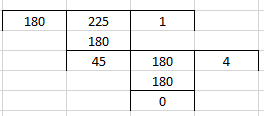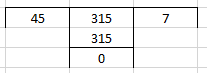Since last division is 45

∴H.C.F. of 180, 225 and 315 = 45

6. Show that 45 and 56 are co-prime numbers.

Solution:

The H.C.F. of two co-prime numbers is always 1

Using division method, the H.C.F. of 45 and 56 are shown below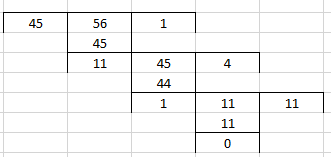Here, last division is 1

H.C.F. of 45 and 56 = 1

∴45 and 56 are co-prime numbers

7. Out of 15, 16, 21 and 28, find out all the pairs of co-prime numbers.

Solution:

15 and 16, 15 and 21, 15 and 28, 16 and 21, 16 and 28 and 21 and 28 will be the possible pairs

We know that the H.C.F. of two co-prime numbers is always 1

Using division method, the H.C.F. of pair 15 and 16 is given below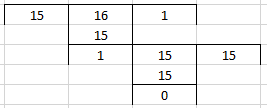Hence, H.C.F. of 15 and 16 = 1

Using division method, the H.C.F. of pair 15 and 21 is given below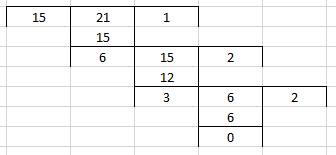Hence, H.C.F. of 15 and 21= 3

Using division method, the H.C.F. of pair 15 and 28 is given below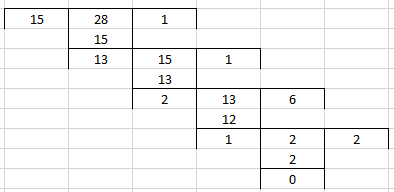Hence, H.C.F. of 15 and 28 = 1

Using division method, the H.C.F. of pair 16 and 21 is given below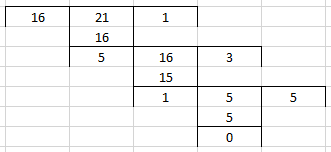Hence, H.C.F. of 16 and 21 = 1

Using division method, the H.C.F. of 16 and 28 is given below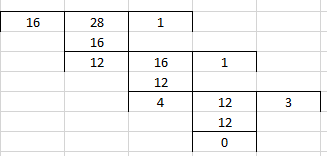Hence, H.C.F. of 16 and 28 = 4

Using division method, the H.C.F. of 21 and 28 is given below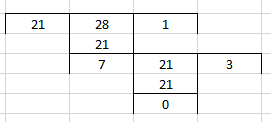Hence, H.C.F. of 21 and 28 = 7

∴ The pairs 15 and 16, 15 and 28 and 16 and 21 are co-prime numbers

8. Find the greatest number that will divide 93, 111 and 129, leaving remainder 3 in each case.

Solution:

First, decrease the leaving remainder 3 from numbers 93, 111 and 129 to find the required number

93 – 3 = 90

111 – 3 = 108

129 – 3 = 126

In each case, the H.C.F. of 90, 108 and 126 will be the greatest number that will divide 93, 111 and 129 leaving remainder 3

Using division method, the H.C.F. of 90, 108 and 126 is given below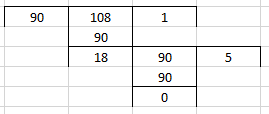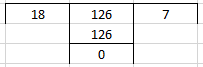Since, last division is 18

∴H.C.F. of 90, 108 and 126 is 18

Hence, the greatest number that will divide 93, 111 and 129 is 18